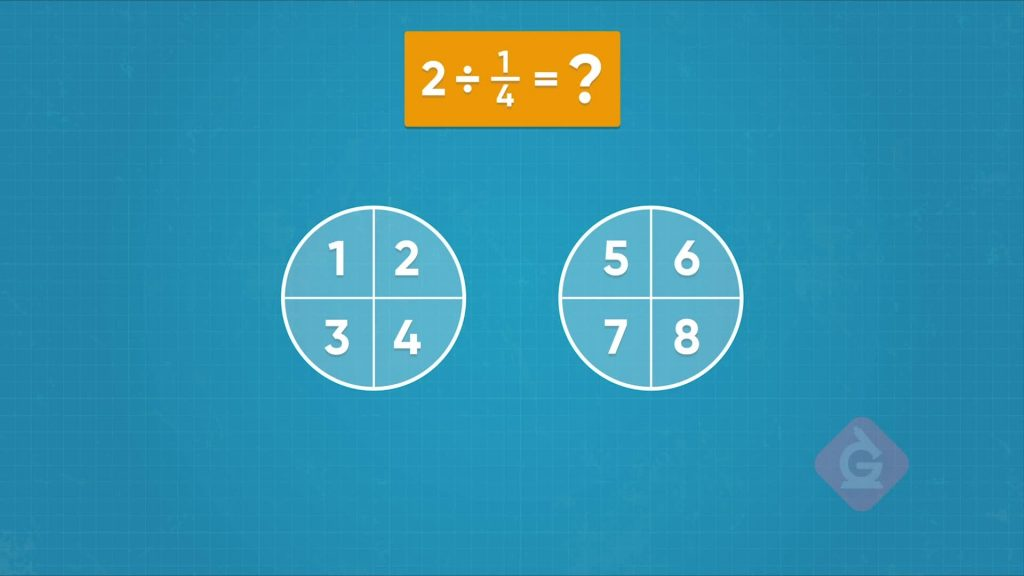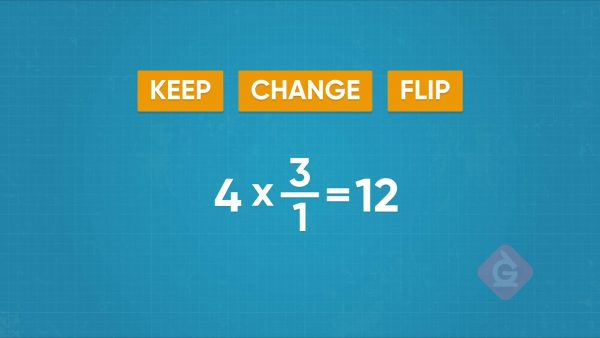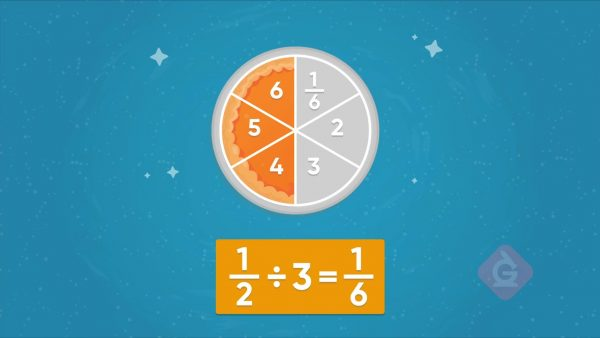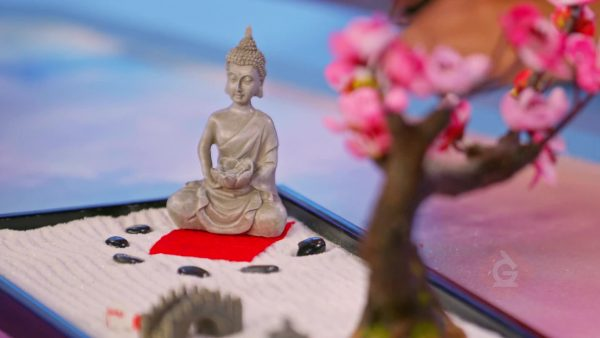Division with Unit Fractions & Whole Numbers | 3rd, 4th, & 5th Grades
1%
It was processed successfully!WHAT IS DIVIDING WHOLE NUMBERS BY UNIT FRACTIONS?

To do this, you will apply and extend your previous understandings of division to divide unit fractions by whole numbers and whole numbers by unit fractions.

To better understand division with unit fractions & whole numbers…

WHAT IS DIVIDING WHOLE NUMBERS BY UNIT FRACTIONS?. To do this, you will apply and extend your previous understandings of division to divide unit fractions by whole numbers and whole numbers by unit fractions. To better understand division with unit fractions & whole numbers…

## LET’S BREAK IT DOWN!

### SlimeLet’s say you want to make some slime. You need [ggfrac]1/4[/ggfrac] cup of glue for each batch. You have 2 cups of glue. You want to find out how many batches of slime you can make. You can write a division equation to show what you are trying to find: 2 ÷ [ggfrac]1/4[/ggfrac] = ? You can draw two circles to represent the 2 cups of glue. Then partition each circle into four equal parts. Each equal part represents [ggfrac]1/4[/ggfrac] of the circle, or [ggfrac]1/4[/ggfrac] cup. Count the number of one-fourths. Each fourth represents one batch of slime that you can make. You can make 8 batches of slime. Try this one yourself. You are mixing blue and yellow paint to create green paint. For each batch of paint, you need [ggfrac]1/3[/ggfrac] cup of yellow paint. You have 3 cups of yellow paint. How many batches of green paint can you make with that amount of yellow paint?

Slime Let’s say you want to make some slime. You need [ggfrac]1/4[/ggfrac] cup of glue for each batch. You have 2 cups of glue. You want to find out how many batches of slime you can make. You can write a division equation to show what you are trying to find: 2 ÷ [ggfrac]1/4[/ggfrac] = ? You can draw two circles to represent the 2 cups of glue. Then partition each circle into four equal parts. Each equal part represents [ggfrac]1/4[/ggfrac] of the circle, or [ggfrac]1/4[/ggfrac] cup. Count the number of one-fourths. Each fourth represents one batch of slime that you can make. You can make 8 batches of slime. Try this one yourself. You are mixing blue and yellow paint to create green paint. For each batch of paint, you need [ggfrac]1/3[/ggfrac] cup of yellow paint. You have 3 cups of yellow paint. How many batches of green paint can you make with that amount of yellow paint?

### Guitar StringsLet’s say you have 4 yards of fishing line you can use to make guitar strings. Each guitar string should be [ggfrac]1/3[/ggfrac] yard long. So, you can use 4 ÷ [ggfrac]1/3[/ggfrac] to find how many guitar strings you can make. You can draw 4 rectangles and divide each rectangle into 3 pieces (thirds). There are 12 thirds in all. You can make 12 guitar strings with the line. You notice that when you're finding the total number of thirds, the total is the same as the whole number TIMES the denominator of the unit fraction. Division and multiplication are opposite operations, like addition and subtraction. You can use multiplication to solve this division problem. To do that you KEEP 4 the same, CHANGE the sign to ×, and FLIP the [ggfrac]1/3[/ggfrac]. After you have done this, the new equation is 4 × [ggfrac]3/1[/ggfrac] , or 4 × 3. 4 × 3 = 12. That means that 4 ÷ [ggfrac]1/3[/ggfrac] is 12, so you can make 12 guitar strings. To find 6 ÷ [ggfrac]1/2[/ggfrac] , you can find 6 × [ggfrac]2/1[/ggfrac], or 6 × 2 = 12. Try this one yourself. For an art piece, you need one piece of ribbon that is [ggfrac]1/8[/ggfrac] inch long. You have 4 inches of ribbon. How many art pieces can you make with the amount of ribbon you have?

Guitar Strings Let’s say you have 4 yards of fishing line you can use to make guitar strings. Each guitar string should be [ggfrac]1/3[/ggfrac] yard long. So, you can use 4 ÷ [ggfrac]1/3[/ggfrac] to find how many guitar strings you can make. You can draw 4 rectangles and divide each rectangle into 3 pieces (thirds). There are 12 thirds in all. You can make 12 guitar strings with the line. You notice that when you're finding the total number of thirds, the total is the same as the whole number TIMES the denominator of the unit fraction. Division and multiplication are opposite operations, like addition and subtraction. You can use multiplication to solve this division problem. To do that you KEEP 4 the same, CHANGE the sign to ×, and FLIP the [ggfrac]1/3[/ggfrac]. After you have done this, the new equation is 4 × [ggfrac]3/1[/ggfrac] , or 4 × 3. 4 × 3 = 12. That means that 4 ÷ [ggfrac]1/3[/ggfrac] is 12, so you can make 12 guitar strings. To find 6 ÷ [ggfrac]1/2[/ggfrac] , you can find 6 × [ggfrac]2/1[/ggfrac], or 6 × 2 = 12. Try this one yourself. For an art piece, you need one piece of ribbon that is [ggfrac]1/8[/ggfrac] inch long. You have 4 inches of ribbon. How many art pieces can you make with the amount of ribbon you have?

### PieLet’s say you have [ggfrac]1/2[/ggfrac] of a pie. You want to partition it into 3 equal pieces for 3 people. What fraction of the whole pie are the new pieces? Find [ggfrac]1/2[/ggfrac] ÷ 3. You can draw a circle to represent a whole pie and then draw a line to split the circle in half. Half of this circle represents the [ggfrac]1/2[/ggfrac] of a pie you start with. Now, partition that [ggfrac]1/2[/ggfrac] piece into 3 equal parts. When you do that, you can see that each smaller piece represents [ggfrac]1/6[/ggfrac] of the pie. That means that [ggfrac]1/2[/ggfrac] ÷ 3 = [ggfrac]1/6[/ggfrac]. Each of the 3 people get [ggfrac]1/6[/ggfrac] of a pie. Try this one yourself. You have [ggfrac]1/4[/ggfrac] of a piece of round cake. You want to partition that piece into 2 equal pieces. What fraction of the whole cake is each small piece?

Pie Let’s say you have [ggfrac]1/2[/ggfrac] of a pie. You want to partition it into 3 equal pieces for 3 people. What fraction of the whole pie are the new pieces? Find [ggfrac]1/2[/ggfrac] ÷ 3. You can draw a circle to represent a whole pie and then draw a line to split the circle in half. Half of this circle represents the [ggfrac]1/2[/ggfrac] of a pie you start with. Now, partition that [ggfrac]1/2[/ggfrac] piece into 3 equal parts. When you do that, you can see that each smaller piece represents [ggfrac]1/6[/ggfrac] of the pie. That means that [ggfrac]1/2[/ggfrac] ÷ 3 = [ggfrac]1/6[/ggfrac]. Each of the 3 people get [ggfrac]1/6[/ggfrac] of a pie. Try this one yourself. You have [ggfrac]1/4[/ggfrac] of a piece of round cake. You want to partition that piece into 2 equal pieces. What fraction of the whole cake is each small piece?

### Zen GardenLet’s say you are making desktop zen gardens. You have a ribbon that is [ggfrac]1/4[/ggfrac] yard long. You want to cut this piece of ribbon into 3 equal pieces, to use one of those pieces in each garden. You want to know how long the pieces are. To find out, you need to find [ggfrac]1/4[/ggfrac] ÷ 3. Use the KEEP CHANGE FLIP strategy to solve. You keep 14 the same, change the division sign to a multiplication sign, and flip 3, or [ggfrac]3/1[/ggfrac], to [ggfrac]1/3[/ggfrac]. [ggfrac]1/4[/ggfrac] × [ggfrac]1/3[/ggfrac]= [ggfrac]1/12[/ggfrac] . Each piece is [ggfrac]1/12[/ggfrac] yards long. Try this one yourself. You have [ggfrac]1/3[/ggfrac] foot of ribbon. You want to cut it into 2 equal pieces. What is the length of each smaller piece?

Zen Garden Let’s say you are making desktop zen gardens. You have a ribbon that is [ggfrac]1/4[/ggfrac] yard long. You want to cut this piece of ribbon into 3 equal pieces, to use one of those pieces in each garden. You want to know how long the pieces are. To find out, you need to find [ggfrac]1/4[/ggfrac] ÷ 3. Use the KEEP CHANGE FLIP strategy to solve. You keep 14 the same, change the division sign to a multiplication sign, and flip 3, or [ggfrac]3/1[/ggfrac], to [ggfrac]1/3[/ggfrac]. [ggfrac]1/4[/ggfrac] × [ggfrac]1/3[/ggfrac]= [ggfrac]1/12[/ggfrac] . Each piece is [ggfrac]1/12[/ggfrac] yards long. Try this one yourself. You have [ggfrac]1/3[/ggfrac] foot of ribbon. You want to cut it into 2 equal pieces. What is the length of each smaller piece?

## DIVISION WITH UNIT FRACTIONS & WHOLE NUMBERS VOCABULARY

Division
Finding how many equal groups or how many in each group.

Whole number
The counting numbers: 0, 1, 2, 3, 4, ...
Fraction
A symbol that describes parts of a whole that is divided into equal parts. For example, [ggfrac]1/3[/ggfrac] or [ggfrac]2/3[/ggfrac].
Unit fraction
A fraction with a numerator of 1. For example, [ggfrac]1/5[/ggfrac] , [ggfrac]1/2[/ggfrac] or [ggfrac]1/10[/ggfrac]
Equation
A mathematical statement that uses an equal sign to show that two expressions are equal. For example, 3 × 4 = 12 or 16 ÷ 2 = 4 × 2.
Partition
Splitting into parts. For example, a circle can be partitioned into fourths.
Combining groups of equal size. You can think of multiplication as repeated addition.
An operation that undoes another operation. Division and multiplication are opposite operations.

## DIVISION WITH UNIT FRACTIONS & WHOLE NUMBERS DISCUSSION QUESTIONS

### You need [ggfrac]1/2[/ggfrac] cup of milk to make your favorite cake. You have 3 cups of milk. Describe a model that represents this situation and can help you find how many cakes you can make.

You need to solve the equation 3 ÷ [ggfrac]1/2[/ggfrac] = ? Draw 3 circles to represent the 3 cups of milk. Each circle is divided in half and each half represents the [ggfrac]1/2[/ggfrac] cup of milk you need for each cake. You can count to find the number of half cups of milk you have. You have 6 half cups of milk. You can make 6 cakes.

### You have a board that is 2 yards long and just the right width for the floor of the bird houses you are building. You need the length of each board to be 14 yard. How many birdhouse floors can you make from this board?

I need to find the answer to 2 ÷ [ggfrac]1/4[/ggfrac] = ? First, I convert the division problem into a multiplication problem: 2 × 4. 2 × 4 = 8. I can make 8 birdhouses with the 2-yard-long wood board.

### You have [ggfrac]1/3[/ggfrac] of a piece of pie. You want to divide that piece into 2 equal pieces to share. What fraction of the pie is each small piece? Write the equation you need to solve, draw a model to solve, and answer the question.

[ggfrac]1/3[/ggfrac] ÷ 2 = [ggfrac]1/6[/ggfrac] . Each small piece is [ggfrac]1/6[/ggfrac] of the pie.

### You have a piece of ribbon that is [ggfrac]1/3[/ggfrac] yard long. You cut it into 2 equal pieces. What is the length of each piece?

[ggfrac]1/6[/ggfrac] yard.

### You have 8 circular cakes. You cut each cake into fourths. Delroy says you have 2 one-fourth pieces because 8 ÷ 4 is 2. Is Delroy correct?

Delroy is not correct. To find the answer, you need to solve the equation 8 ÷ [ggfrac]1/4[/ggfrac] = ? To divide 8 by [ggfrac]1/4[/ggfrac], you change the division equation into the multiplication equation 8 × 4 = ? 8 × 4 = 32, so there are 32 one-fourth pieces.

X

## Success

We’ve sent you an email with instructions how to reset your password.
Ok